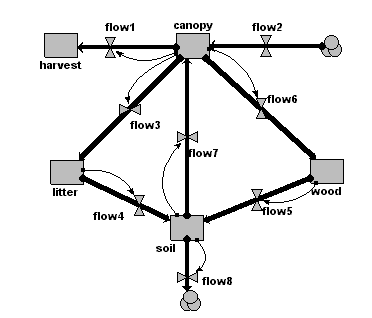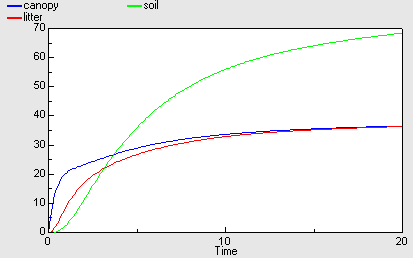# Nitrogen dynamics

## Simple teaching model of forest nitrogen dynamics

ModelId:
besn1
SimileVersion:
3.1+

### This is a very simple, generic model of nitrogen dynamics in a forest. It was developed as a teaching model, with very simple flow equations and arbitrary parameter values.Category:
Specific
Equations:

Compartments:
canopy: initial value = 0
harvest: initial value = 0
litter: initial value = 0
wood: initial value = 0
soil: initial value = 0

Flows:
flow1 = 0.9*canopy
flow2 = 50
flow3 = 0.9*canopy
flow4 = 0.9*litter
flow5 = 0.01*wood
flow6 = 0.5*canopy
flow7 = 0.5*soil
flow8 = 0.01*soil

Results:Tags:
Model tags: